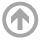Daniel BatesI am a numerical algebraic geometer in the math department at the US Naval Academy. I have various research interests, though much of my work so far is in some way related to the field of numerical algebraic geometry (numerical methods for computing quantities and structures related to polynomial systems). Here is a broader (but not complete) list of some areas that interest me

• Numerical algebraic geometry
• Numerical analysis
• Algebraic geometry
• Scientific computing (primarily software development)

You might know me from the decade I spent in the Colorado State University Math Department.  As I set up my new webpages here at the Academy, please refer back to my CSU webpage for preprints, primers on my area, and various other research-related efforts.